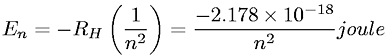Equations > Chemistry > Atomic Structure > Relationship between Energy and Principal Quantum Number

### Relationship between Energy and Principal Quantum NumberLatex Code:

MathML Code:

 ${E}_{n}=-{R}_{H}\left(\frac1{n}^{2}\right)=\frac-2.178×{10}^{-18}{n}^{2}\mathrm{joule}$

MathType 5.0: